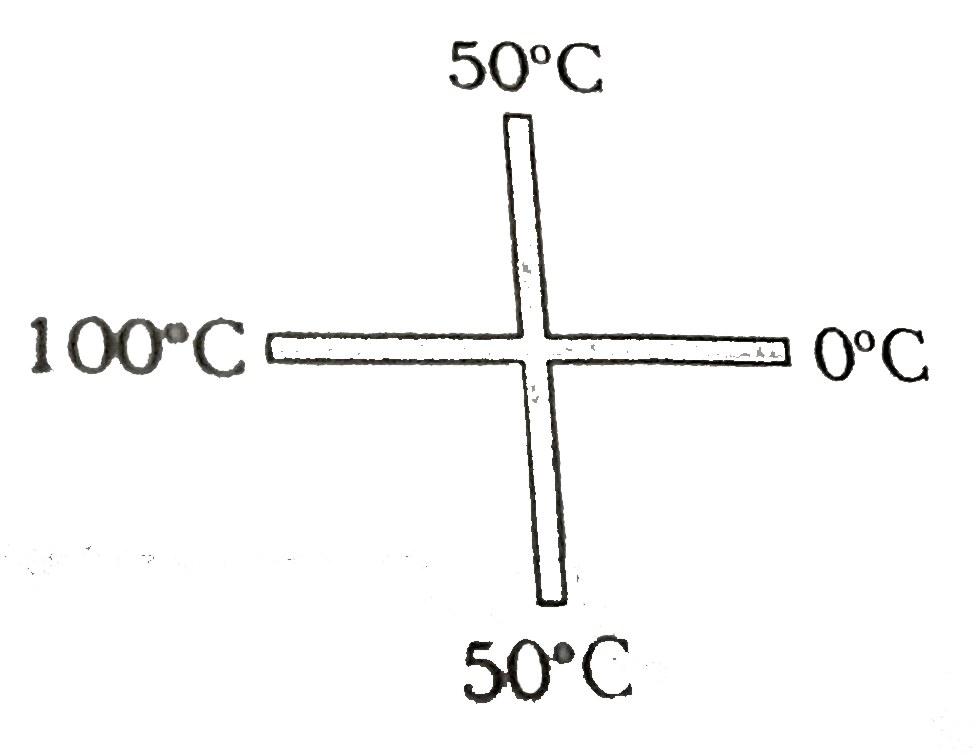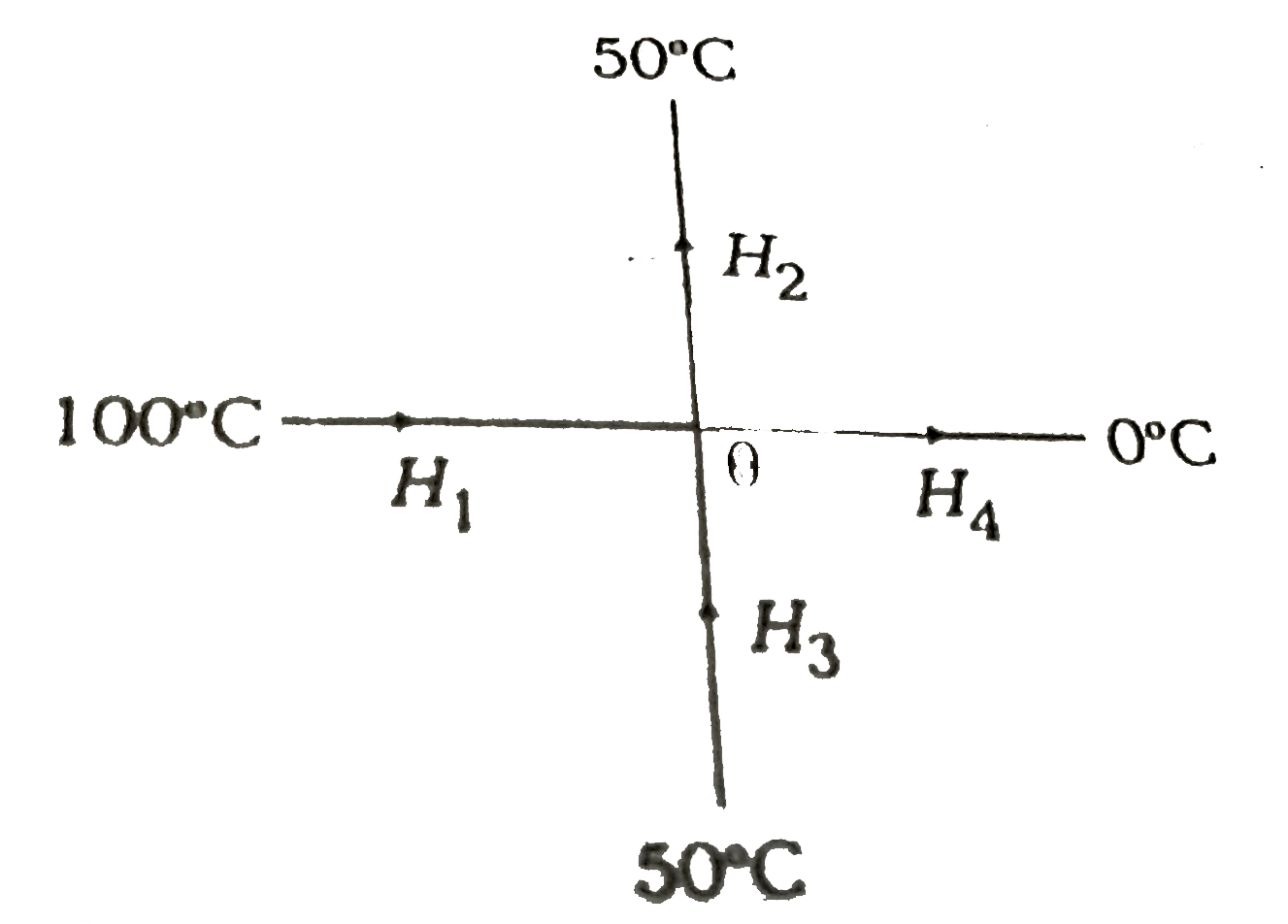# Two similar rods are joined as shown in figure. Then temperature of junction is (assume no heat loss through lateral surface of rod and temperatures a

97 views
in Physics
closed
Two similar rods are joined as shown in figure. Then temperature of junction is (assume no heat loss through lateral surface of rod and temperatures at the ends are shown in steady state )A. 50^(@)C
B. 75^(@)C
C. 66.6^(@)C
D. 33.3^(@)C

by (90.6k points)
selected

Let theta be the temperature of junction.H_(1),H_(2),H_(3) and H_(4) be the heat currents. Then and
H_(1)=H_(2)+H_(3)+H_(4)
or (100-theta)/R=(theta-50)/R+(theta-50)/R+(theta-0)/R
(R = Thermal resistance of each part of two rods)
Solving these two equations, we get
theta= 50^(@)C